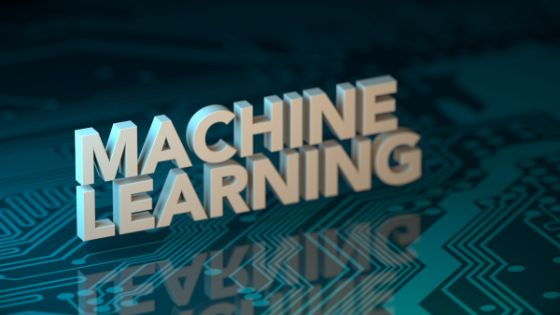Performance metrics are an essential part of the machine learning process. It is the indicator that identifies whether a machine learning process is progressing in the right direction or not.

Machine learning model monitoring metrics have a performance component that reduces the performance of the process. It is universal for all machine learning models like the linear regression or SOTA technique.The machine learning activity or process can be classified into either Regression or Classification. There are several metrics for both approaches. One important thing at this stage is to understand how the model views the sample data for learning.

The performance of the model can also be done through the loss functions. However, they are different from the metrics.

The metrics are used to measure the model’s performance during the training and testing phase. They do not need to be differentiable. Whereas loss functions are used to train a model using an optimization process. They are usually differentiable.

## The Regression Metrics

Regression metrics have a continuous output, and the metric calculates the distance between predicted and ground truth.

• Mean Squared Error (MSE)
• Mean Absolute Error (MAE)
• Root Mean Squared Error (RMSE)
• R² (R-Squared)

These are some of the famous metrics in Regression Metrics.

### Mean Squared Error (MSE)

The Mean Squared Error (MSE) essentially calculates and finds the average squared differences between the value predicted by the regression model and the target value. The Mean Squared Error is differentiable.

As a result, it can optimize better. It squares the errors. This essentially leads to an overestimation of the negative efficacy of the model.

### Mean Absolute Error (MAE)

Mean Absolute Error (MAE) is the average value of the difference between the ground truth and the predicted value. It is more robust. It gives the distance between the predictions and the actual values. The error interpretation aligns perfectly with the original degree of the variable. It is non-differentiable.

### Root Mean Squared Error (RMSE)

The RMSE (Root Mean Squared Error) squares the difference between the target and predicted values. It retains the differential property of the model. It penalizes the errors by square rooting them. The error interpretation can be made smoothly in this metric.

### R² Coefficient of determination

The R² Coefficient of determination works as a post metric. It calculates using other metrics. If the sum of the squared error of the regression line is small, then the R2 will be closed.

### Classification metrics

The classification Metric is one of the most widely researched machine learning model monitoring metrics. Almost all cases of industrial environments and production use cases are present. Face recognition, speech recognition, text classification, and more. The list is never-ending.

All classification models have a separate discrete output. The Classification Metrics can evaluate the performance of a model and can tell how bad or good the classification is in real-time.

• Confusion Matrix
• Accuracy
• F1-score
• Precision and Recall
• AU-ROC

These are some of the famous metrics in Classification Metrics.

#### To Conclude

This article attempts to elaborate on the importance of performance metrics in machine learning model evaluation. The above-mentioned machine learning model monitoring metrics help in understanding the efficacy of the machine learning model.

Therefore, it is suggested to keep experimenting with the machine learning models and their performance metrics.